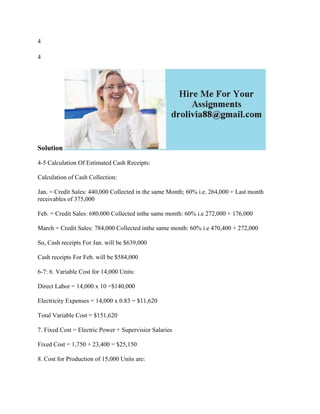Diese Präsentation wurde erfolgreich gemeldet.

# 4 4 Solution4-5 Calculation Of Estimated Cash Receipts- Calcul.docx

Anzeige
Anzeige
Anzeige
Anzeige
Anzeige
Anzeige
Anzeige
Anzeige
Anzeige
Anzeige
Anzeige×

1 von 2 Anzeige

# 4 4 Solution4-5 Calculation Of Estimated Cash Receipts- Calcul.docx

4
Solution
4-5 Calculation Of Estimated Cash Receipts:
Calculation of Cash Collection:
Jan. = Credit Sales: 440,000 Collected in the same Month; 60% i.e. 264,000 + Last month receivables of 375,000
Feb. = Credit Sales: 680,000 Collected inthe same month: 60% i.e 272,000 + 176,000
March = Credit Sales: 784,000 Collected inthe same month: 60% i.e 470,400 + 272,000
So, Cash receipts For Jan. will be \$639,000
Cash receipts For Feb. will be \$584,000
6-7: 6. Variable Cost for 14,000 Units:
Direct Labor = 14,000 x 10 =\$140,000
Electricity Expenses = 14,000 x 0.83 = \$11,620
Total Variable Cost = \$151,620
7. Fixed Cost = Electric Power + Supervisior Salaries
Fixed Cost = 1,750 + 23,400 = \$25,150
8. Cost for Production of 15,000 Units are:

.

4
Solution
4-5 Calculation Of Estimated Cash Receipts:
Calculation of Cash Collection:
Jan. = Credit Sales: 440,000 Collected in the same Month; 60% i.e. 264,000 + Last month receivables of 375,000
Feb. = Credit Sales: 680,000 Collected inthe same month: 60% i.e 272,000 + 176,000
March = Credit Sales: 784,000 Collected inthe same month: 60% i.e 470,400 + 272,000
So, Cash receipts For Jan. will be \$639,000
Cash receipts For Feb. will be \$584,000
6-7: 6. Variable Cost for 14,000 Units:
Direct Labor = 14,000 x 10 =\$140,000
Electricity Expenses = 14,000 x 0.83 = \$11,620
Total Variable Cost = \$151,620
7. Fixed Cost = Electric Power + Supervisior Salaries
Fixed Cost = 1,750 + 23,400 = \$25,150
8. Cost for Production of 15,000 Units are:

.

Anzeige
Anzeige

### 4 4 Solution4-5 Calculation Of Estimated Cash Receipts- Calcul.docx

1. 1. 4 4 Solution 4-5 Calculation Of Estimated Cash Receipts: Calculation of Cash Collection: Jan. = Credit Sales: 440,000 Collected in the same Month; 60% i.e. 264,000 + Last month receivables of 375,000 Feb. = Credit Sales: 680,000 Collected inthe same month: 60% i.e 272,000 + 176,000 March = Credit Sales: 784,000 Collected inthe same month: 60% i.e 470,400 + 272,000 So, Cash receipts For Jan. will be \$639,000 Cash receipts For Feb. will be \$584,000 6-7: 6. Variable Cost for 14,000 Units: Direct Labor = 14,000 x 10 =\$140,000 Electricity Expenses = 14,000 x 0.83 = \$11,620 Total Variable Cost = \$151,620 7. Fixed Cost = Electric Power + Supervisior Salaries Fixed Cost = 1,750 + 23,400 = \$25,150 8. Cost for Production of 15,000 Units are:
2. 2. Opening Receivables Sales Sales on Account (80%) Cash Collected Closing Receivables Jan 375,000 550,000 440,000 639,000 176,000 Feb. 176,000 850,000 680,000 584,000 272,000 March 272,000 980,000 784,000 742,400 470,400